Lec 10 - RREF and Rank

Wednesday, January 25, 2012

2:16 AM

Recall:

A system of linear equations is homogeneous if the RHS contains only zeros.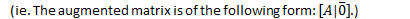Eg

The only solution of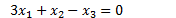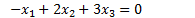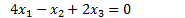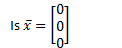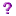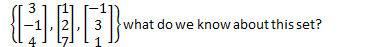We know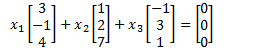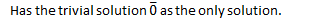Thus the set is linearly independent.

Eg.

Solve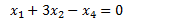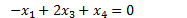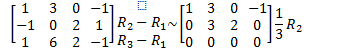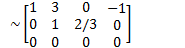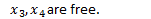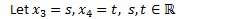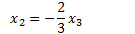The solution set has vector equation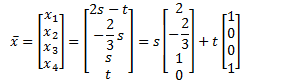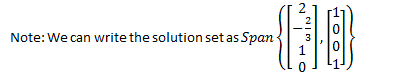Theorem: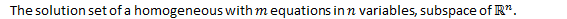Rank

Definition

The rank of a matrix is the number of leading ones in the RREF of the matrix.Eg.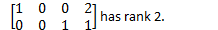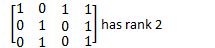Theorem1.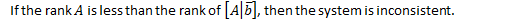1.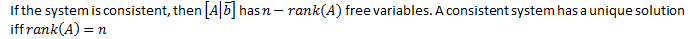1.Proof: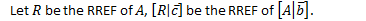1.1.1.Eg.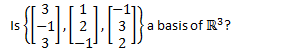First, is the set linear independent?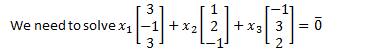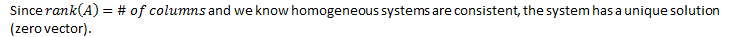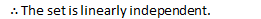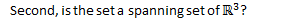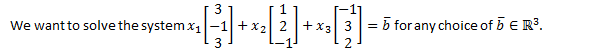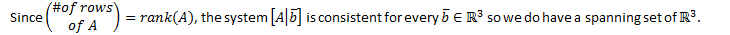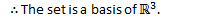Proof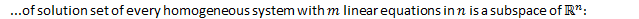Note that every homogeneous system is consistent.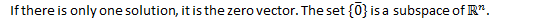If there is an infinite number of solutions, let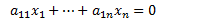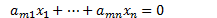Be the homogeneous system.

Apply the Subspace Test:

The solution set is non-empty since the system is consistent.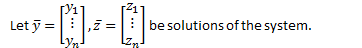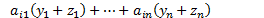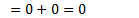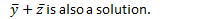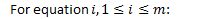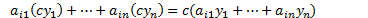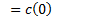Created by Tim Pei with Microsoft OneNote 2010
One place for all your notes and information